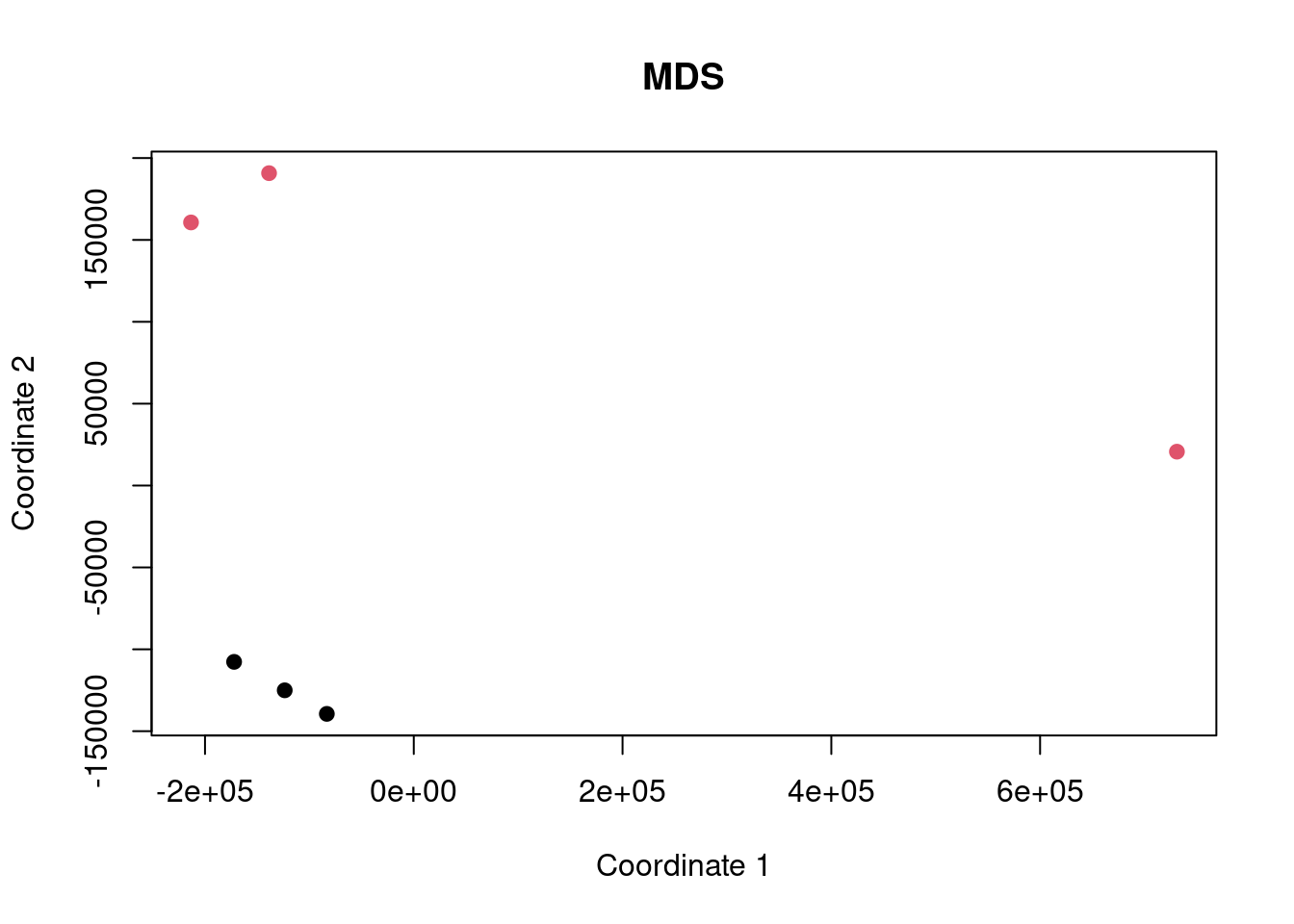## Intro

Here we are performing an analysis of some gene expression data to demonstrate the difference between ORA and FCS methods and to highlight the differences caused by improper background gene set use.

The dataset being used is SRP253951 and we are comparing A549 cells with and without infection with SARS-CoV-2.

Data are obtained from http://dee2.io/

``````suppressPackageStartupMessages({
library("getDEE2")
library("DESeq2")
library("clusterProfiler")
library("mitch")
library("kableExtra")
library("eulerr")
})``````

## Get expression data

I’m using some RNA-seq data looking at the difference in transcriptome caused by SARS-CoV-2 infection.

``````name="SRP253951"
samplesheet <- mdat[grep("SRP253951",mdat\$SRP_accession),]

samplesheet <- mdat[which(mdat\$SRX_accession %in% c("SRX8089264","SRX8089265","SRX8089266","SRX8089267","SRX8089268","SRX8089269")),]

samplesheet\$trt <- factor(c(0,0,0,1,1,1))
s1 <- samplesheet

s1 %>% kbl(caption = "sample sheet") %>% kable_paper("hover", full_width = F)``````
sample sheet
SRR_accession QC_summary SRX_accession SRS_accession SRP_accession Sample_name GEO_series Library_name trt
229503 SRR11517674 PASS SRX8089264 SRS6456133 SRP253951 GSM4462336 GSE147507 0
229504 SRR11517675 PASS SRX8089265 SRS6456134 SRP253951 GSM4462337 GSE147507 0
229505 SRR11517676 PASS SRX8089266 SRS6456135 SRP253951 GSM4462338 GSE147507 0
229506 SRR11517677 PASS SRX8089267 SRS6456136 SRP253951 GSM4462339 GSE147507 1
229507 SRR11517678 PASS SRX8089268 SRS6456137 SRP253951 GSM4462340 GSE147507 1
229508 SRR11517679 PASS SRX8089269 SRS6456139 SRP253951 GSM4462341 GSE147507 1
``w<-getDEE2("hsapiens",samplesheet\$SRR_accession,metadata=mdat,legacy = TRUE)``
``````## For more information about DEE2 QC metrics, visit
##     https://github.com/markziemann/dee2/blob/master/qc/qc_metrics.md``````
``````x<-Tx2Gene(w)
x <- x\$Tx2Gene

# save the genetable for later
gt<-w\$GeneInfo[,1,drop=FALSE]
gt\$accession<-rownames(gt)

# counts
x1<-x[,which(colnames(x) %in% samplesheet\$SRR_accession)]``````

Here show the number of genes in the annotation set, and those detected above the detection threshold.

``````# filter out lowly expressed genes
x1<-x1[which(rowSums(x1)/ncol(x1)>=(10)),]
nrow(x)``````
``##  39297``
``nrow(x1)``
``##  15182``

Now multidimensional scaling (MDS) plot to show the correlation between the datasets. If the control and case datasets are clustered separately, then it is likely that there will be many differentially expressed genes with FDR<0.05.

``plot(cmdscale(dist(t(x1))), xlab="Coordinate 1", ylab="Coordinate 2", pch=19, col=s1\$trt, main="MDS")``## Differential expression

Now run DESeq2 for control vs case.

``y <- DESeqDataSetFromMatrix(countData = round(x1), colData = s1, design = ~ trt)``
``## converting counts to integer mode``
``y <- DESeq(y)``
``## estimating size factors``
``## estimating dispersions``
``## gene-wise dispersion estimates``
``## mean-dispersion relationship``
``## final dispersion estimates``
``## fitting model and testing``
``````de <- results(y)
de<-as.data.frame(de[order(de\$pvalue),])
rownames(de)<-sapply(strsplit(rownames(de),"\\."),"[[",1)
head(de) %>% kbl() %>% kable_paper("hover", full_width = F)``````
baseMean log2FoldChange lfcSE stat pvalue padj
ENSG00000113739 3523.734 4.004593 0.1012568 39.54888 0 0
ENSG00000176153 8851.309 -2.785312 0.0820808 -33.93378 0 0
ENSG00000058085 5767.111 3.699041 0.1148334 32.21223 0 0
ENSG00000169710 6917.854 -2.259158 0.0703230 -32.12547 0 0
ENSG00000170421 41561.919 -2.233482 0.0742019 -30.10007 0 0
ENSG00000100297 2476.082 -2.364383 0.0802583 -29.45965 0 0

Now let’s have a look at some of the charts showing differential expression. In particular, an MA plot and volcano plot.

``````maplot <- function(de,contrast_name) {
sig <-subset(de, padj < 0.05 )
up <-rownames(subset(de, padj < 0.05 & log2FoldChange > 0))
dn <-rownames(subset(de, padj < 0.05 & log2FoldChange < 0))
GENESUP <- length(up)
GENESDN <- length(dn)
DET=nrow(de)
SUBHEADER = paste(GENESUP, "up, ", GENESDN, "down", DET, "detected")
ns <-subset(de, padj > 0.05 )
plot(log2(de\$baseMean),de\$log2FoldChange,
xlab="log2 basemean", ylab="log2 foldchange",
pch=19, cex=0.5, col="dark gray",
main=contrast_name, cex.main=0.7)
points(log2(sig\$baseMean),sig\$log2FoldChange,
pch=19, cex=0.5, col="red")
}

make_volcano <- function(de,name) {